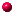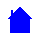## ELET1210 - Digital ElectronicsLECTURE NOTESBasic GatesCompound GatesRules of Boolean AlgebraProofs (simplification) of Compound RulesDe Morgan's Theorem visually explainedDe Morgan's Theorem worked examplesDifferent ways of writing Boolean functions/expressionsSimplification of SOP expressionsSimplification of POS expressionsSimplification of POS - worked examplethe minterms on Karnaugh mapsRules of adjacent squares and 4-variable Karnaugh mapsSimple functions on 2 and 3-variable Karnaugh mapsStep by step HELP to Karnaugh maps (part 1)Step by step HELP to Karnaugh maps (part 2)Tutorial examples using Karnaugh mapsHexadecimal, binary and decimal numbersNumber conversionsNegative numbers and codesMathematical addition and subtraction of binary numbersBinary Subtraction & Truth Table (added 5 February 2015)Adders and Subtractors3-bit adderComplement subtraction (Minuend LESS THAN Subtrahend)Complement subtraction (Minuend GREATER THAN Subtrahend)Real Number conversionsDuality and ComplementNAND-NAND and NOR-NORSingle Variables with NAND-NAND and NOR-NOREncoders, Decoders, Multiplexers and DemultiplexersEncoders and DecodersMultiplexers and DemultiplexersParity generation and checkingBCD additionImplementing Functions with a MultiplexerSequential Logic and S-R LatchFlip-Flop TypesGlitches and Master-Slave Flip-FlopsFlip-Flop Excitation TablesFinite State Devices - D type flip-flopsFinite State Devices - JK type flip-flopsDesigning synchronous countersELET1210 Homepage